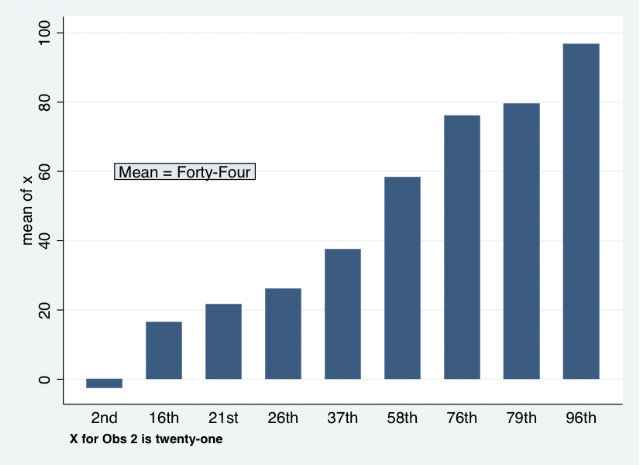A new SSC package to convert numbers to text (-num2words-)

-num2words- has been posted to the SSC Archives.  It is a Stata module to convert numbers to text.  It can convert integers, fractional numbers, and ordinal numbers (e.g., 8 to 8th).  The idea for this program originated from a LaTeX report I was creating that had some code that wrote the text version of numbers into sentences, including writing the proper case text for a number if it started a sentence.  So, the LaTeX file (written via -texdoc- from SSC) had some code like:
****texdoc example
sum x, meanonly
loc totalN "`=_N'"
loc pct1  "`=myvar'"
loc totalN "`r(N)'"
if `totalN'>`lastN' loc change1 "increase"
****texdoc text written:
tex  `totalN' respondents took the survey this month.
tex  There was a `pct1' percent `change1' in respondents who reported using incentive payment dollars....and so on
****

where the macros are defined as:
`totalN' - the total number of relevant respondents (so, loc totalN "`=_N'")
`pct1' - the calculated percent respondents from the dataset (so, loc pct1  "`=myvar'")
`change1' - substitutes the word "increase" in if `pct1' increased from the last survey wave, "decrease" for a decrease and "equal to" if it was the same.
I created -num2words- to ease the process of converting many variables--like those underlying the macros `totalN' and `pct1'--to words/text, so it can change "212 respondents" to "Two-hundred and twelve respondents" or  "25.5 percent" to "twenty-five and 5 tenths percent" (or it can be truncated to just "twenty-five percent" with the "round" option in -num2words-) in the narrative.
You can also automatically change numbers to words for insertion in table or figure titles, notes, etc.
******************fig 1 example
clear
set obs 10
g x = round(runiform()*100, .05)
num2words x, g(x_rounded) round
**graph**
egen mx = mean(x)
num2words mx, round
gr bar x  , over(x2_ordinal, sort(1)) ///
note({bf: X for Obs 2 is `=x_rounded'}) ///
text(60 20 `"Mean = `=mx2'"',  box )
**********************fig 1 exampleFig. 1

You can get -num2words- from the SSC Archives